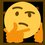# Proof of Mean Value Theorem with Rolle's Theorem

Theorem: if $$f$$ is continuous on $$[a,b]$$ and $$f$$ is differentiable on $$(a,b)$$, then $$\frac{f(b)-f(a)}{b-a}=f^\prime(c)$$ for some $$c$$ in $$(a,b)$$.

Rolle's Theorem: if $g$ is continuous on $[a,b]$, $g$ is differentiable on $(a,b)$ and $g(b)=g(a)$, then there is $c$ in $(a,b)$ such that $g^\prime(c)=0$.

Proof:

$\text{Let }g(x)=f(x)-\left(\frac{f(b)-f(a)}{b-a}\right)x$

\begin{aligned} g(a)&=f(a)-\left(\frac{f(b)-f(a)}{b-a}\right)a\\ &=f(a)-\left(\frac{a}{b-a}\right)f(b)+\left(\frac{a}{b-a}\right)f(a)\\ &=\left(1+\frac{a}{b-a}\right)f(a)-\left(\frac{a}{b-a}\right)f(b)\\ &=\left(\frac{b}{b-a}\right)f(a)-\left(\frac{a}{b-a}\right)f(b) \end{aligned}

\begin{aligned} g(b)&=f(b)-\left(\frac{f(b)-f(a)}{b-a}\right)b\\ &=f(b)-\left(\frac{b}{b-a}\right)f(b)+\left(\frac{b}{b-a}\right)f(a)\\ &=\left(1-\frac{b}{b-a}\right)f(b)+\left(\frac{b}{b-a}\right)f(a)\\ &=-\left(\frac{a}{a-b}\right)f(b)+\left(\frac{b}{b-a}\right)f(a) \end{aligned}

$g(b)=g(a)\Rightarrow g^\prime(c)=0$

\begin{aligned} g(x)&=f(x)-\left(\frac{f(b)-f(a)}{b-a}\right)x\\ g^\prime(x)&=f^\prime(x)-\frac{f(b)-f(a)}{b-a}\\ g^\prime(c)&=f^\prime(c)-\frac{f(b)-f(a)}{b-a}\\ 0&=f^\prime(c)-\frac{f(b)-f(a)}{b-a}\\ \frac{f(b)-f(a)}{b-a}&=f^\prime(c)\quad\blacksquare \end{aligned}Note by Gandoff Tan
1 year, 6 months ago

This discussion board is a place to discuss our Daily Challenges and the math and science related to those challenges. Explanations are more than just a solution — they should explain the steps and thinking strategies that you used to obtain the solution. Comments should further the discussion of math and science.

When posting on Brilliant:

• Use the emojis to react to an explanation, whether you're congratulating a job well done , or just really confused .
• Ask specific questions about the challenge or the steps in somebody's explanation. Well-posed questions can add a lot to the discussion, but posting "I don't understand!" doesn't help anyone.
• Try to contribute something new to the discussion, whether it is an extension, generalization or other idea related to the challenge.

MarkdownAppears as
*italics* or _italics_ italics
**bold** or __bold__ bold
- bulleted- list
• bulleted
• list
1. numbered2. list
1. numbered
2. list
Note: you must add a full line of space before and after lists for them to show up correctly
paragraph 1paragraph 2

paragraph 1

paragraph 2

[example link](https://brilliant.org)example link
> This is a quote
This is a quote
    # I indented these lines
# 4 spaces, and now they show
# up as a code block.

print "hello world"
# I indented these lines
# 4 spaces, and now they show
# up as a code block.

print "hello world"
MathAppears as
Remember to wrap math in $$ ... $$ or $ ... $ to ensure proper formatting.
2 \times 3 $2 \times 3$
2^{34} $2^{34}$
a_{i-1} $a_{i-1}$
\frac{2}{3} $\frac{2}{3}$
\sqrt{2} $\sqrt{2}$
\sum_{i=1}^3 $\sum_{i=1}^3$
\sin \theta $\sin \theta$
\boxed{123} $\boxed{123}$

Sort by:

Hello! My name is diana and im from portugal. I noticed we're the same age, so i was wandering if you learned this at school, because i had never heard of it (and im taking 12th grade math). Is the curriculum different in singapure or did you learn it by yourself?

- 1 year, 5 months ago

I learnt it from the internet. It's uni year 1 stuff.

- 1 year, 5 months ago